Find the derivative r ‘(t) of the vector function r(t). t sin 6t , t2, t cos 7t Part 1 of 4 The derivative of a vector function is obtained

Question

Find the derivative r ‘(t) of the vector function r(t). t sin 6t , t2, t cos 7t Part 1 of 4 The derivative of a vector function is obtained by differentiating each of its components. Thus, if r(t) = f(t), g(t), h(t) , where f, g, and h are differentiable functions, then r'(t) = f ‘(t), g'(t), h'(t) . For r(t) = t sin 6t, t2, t cos 7t , we have f(t) = t sin 6t, which is a product. Using the Product Rule and the Chain Rule, we have

in progress 0
6 months 2021-08-02T18:33:10+00:00 1 Answers 8 views 0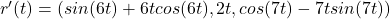Step-by-step explanation:

We need to find the derivative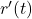of the vector function :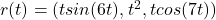In order to find, we are going to differentiate each of its components ⇒

We can write the following ⇒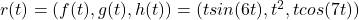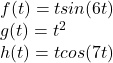Let’s differentiate each function to obtain: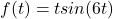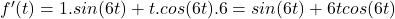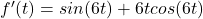Now with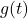: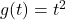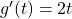With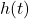: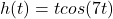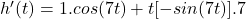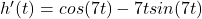Finally we need to complete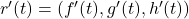with its components :